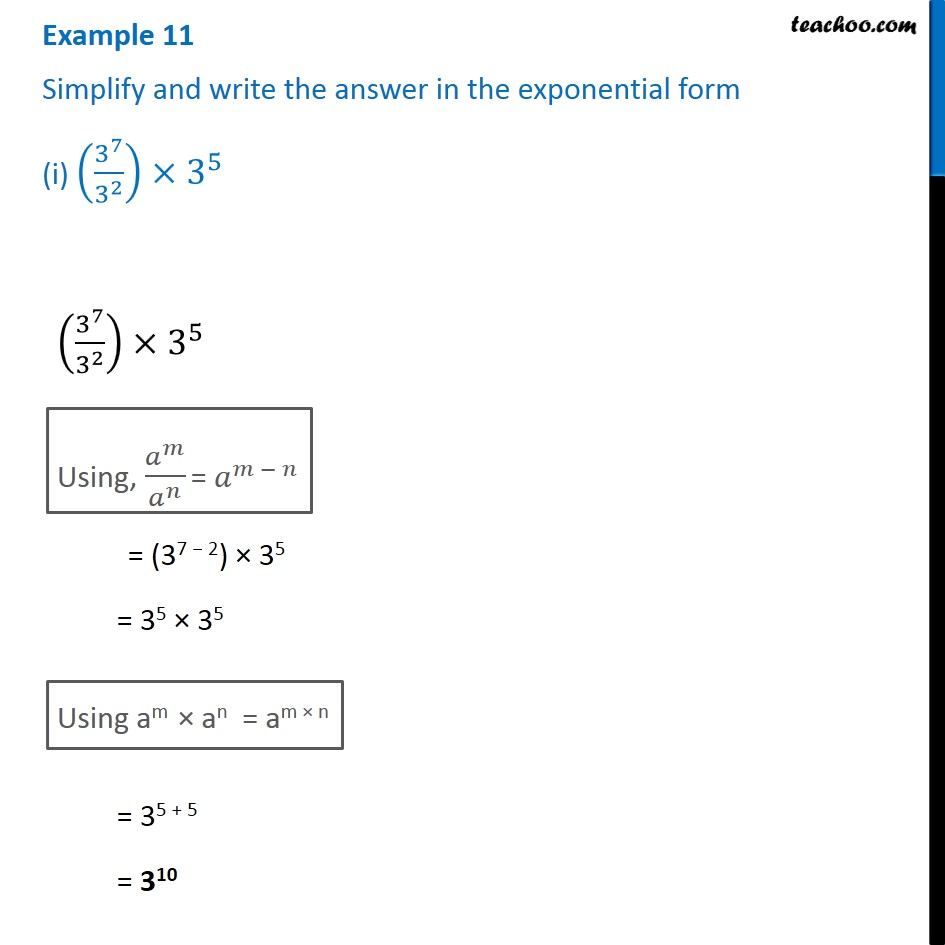1. Chapter 13 Class 7 Exponents and Powers
2. Concept wise
3. Law of exponents

Transcript

Example 11 Simplify and write the answer in the exponential form (i) (3^7/3^2 )×3^5 (3^7/3^2 )×3^5 Using, 𝑎^𝑚/𝑎^𝑛 = 𝑎^(𝑚 − 𝑛) = (37 − 2) × 35 = 35 × 35 Using am × an = am × n = 35 + 5 = 310